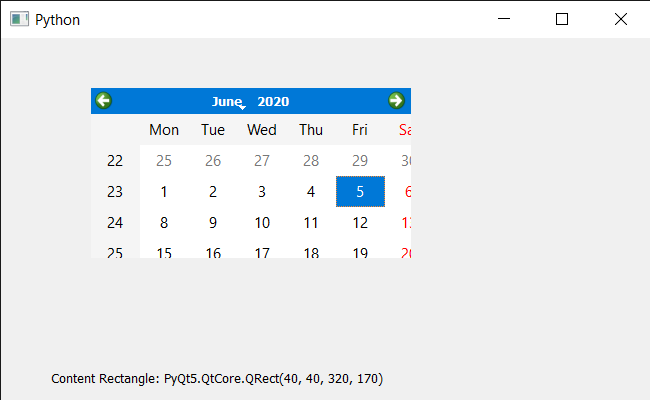Related Articles

# PyQt5 QCalendarWidget – Getting Content Rectangle

• Last Updated : 08 Jun, 2020

In this article we will see how we can get the content rectangle to the QCalendarWidget. Content rectangle is the bounding rectangle of the content, by default content margin value is zero, so content rectangle is equal to the actual bounding rectangle but when margin change it become smaller as content rectangle is the rectangle excluding the margins. We can add margin with the help of `setContentsMargins` method.

In order to do this we will use `contentsRect` method with the QCalendarWidget object.

Syntax : calendar.contentsRect()

Argument : It takes no argument

Return : It return QRect object

Below is the implementation

 `# importing libraries``from` `PyQt5.QtWidgets ``import` `*` `from` `PyQt5 ``import` `QtCore, QtGui``from` `PyQt5.QtGui ``import` `*` `from` `PyQt5.QtCore ``import` `*` `import` `sys`` ` ` ` `class` `Window(QMainWindow):`` ` `    ``def` `__init__(``self``):``        ``super``().__init__()`` ` `        ``# setting title``        ``self``.setWindowTitle(``"Python "``)`` ` `        ``# setting geometry``        ``self``.setGeometry(``100``, ``100``, ``650``, ``400``)`` ` `        ``# calling method``        ``self``.UiComponents()`` ` `        ``# showing all the widgets``        ``self``.show()`` ` `    ``# method for components``    ``def` `UiComponents(``self``):`` ` `        ``# creating a QCalendarWidget object``        ``self``.calender ``=` `QCalendarWidget(``self``)`` ` `        ``# setting geometry to the calender``        ``self``.calender.setGeometry(``50``, ``10``, ``400``, ``250``)`` ` `        ``# setting content margin``        ``self``.calender.setContentsMargins(``40``, ``40``, ``40``, ``40``)`` ` `        ``# creating a label``        ``label ``=` `QLabel(``self``)`` ` `        ``# setting geometry``        ``label.setGeometry(``50``, ``280``, ``420``, ``120``)`` ` `        ``# making it multi line``        ``label.setWordWrap(``True``)`` ` `        ``# getting content Rectangle``        ``value ``=` `self``.calender.contentsRect()`` ` `        ``# setting text to the label``        ``label.setText(``"Content Rectangle: "` `+` `str``(value))`` ` ` ` ` ` ` ` `# create pyqt5 app``App ``=` `QApplication(sys.argv)`` ` `# create the instance of our Window``window ``=` `Window()`` ` ` ` `# start the app``sys.exit(App.``exec``())`

Output :Attention geek! Strengthen your foundations with the Python Programming Foundation Course and learn the basics.

To begin with, your interview preparations Enhance your Data Structures concepts with the Python DS Course. And to begin with your Machine Learning Journey, join the Machine Learning – Basic Level Course

My Personal Notes arrow_drop_up